##### Child pages
• Reducing an employee's Normal Hours per week

## Key

• This line was removed.
• Formatting was changed.
HTML Wrap
class section group
HTML Wrap
class col span_3_of_5

When you reduce an employee's Normal Hours per week, their annual leave calculation rate will increase but the allocation of annual leave will remain the same. This means the employee will appear to be owed more annual leave than they should have.

This support note explains how to reduce an employee's Normal Hours per week, then how to correct their Current Annual Leave Due.

The example we'll use is Bill who is reducing his work hours from 40 to 24 hours per week.

UI Text Box
type note

Before you begin

Print the affected employee's accrued leave report for your records prior to making any adjustments. This report can be accessed by going to Reports > Employee Reports > Employee Holidays.

If you need to increase an employee's normal pay, see Increasing an employee's Normal Hours per week.

UI Expand
title To reduce an employee's Normal Hours per week
1. Go to the Maintenance command centre and click Maintain Employees.
2. Select the applicable employee.
3. Click the Leave Details tab.
4. Enter the required values in the Normal days/week and Normal hours/day fields. This will adjust their Normal Hours per week.

The Normal Hours per week setting is used:

• to allocate the annual leave entitlement for the year. For example an employee working 40 normal hours per week is allocated 160 hours leave at their anniversary date (4 weeks X 40 hours per week=160 hours).
• as a divisor for the calculation of the annual leave rate. The Average Weekly Earnings and the Ordinary Weekly Pay are divided by the Normal Hours per week to calculate the annual leave rate.

## What is the effect of reducing the Normal Hours per week?

As shown below, Bill currently works 8 hours a day, 5 days a week, totalling 40 hours a week. This gives Bill an annual leave allocation balance of 160 hours a year. His current annual leave due rate is \$31 which equates to \$4960 per year (\$31 x 160 hours).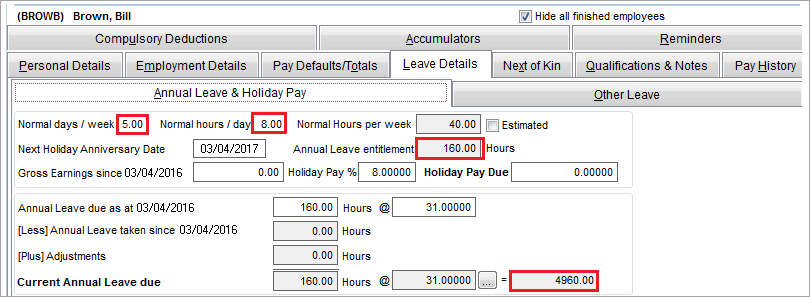Here's what happens when we reduce Bill's days per week from 5 to 3: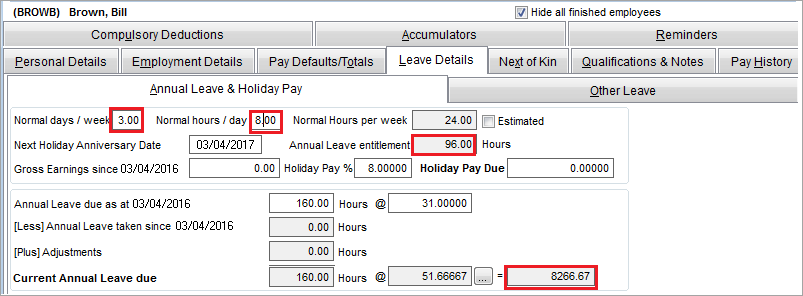This reduces Bill's Annual Leave Entitlement to 96 hours a year, and increases his rate to \$51.66, which is correct. However if no other changes are made, this makes the Current Annual Leave Due \$8266.67 (\$51.66 x 160 hours), an increase of \$3306.67, which is incorrect.

The next task explains how to correct the Current Annual Leave Due.

UI Expand
title To correct the Current Annual Leave Due

To correct the Current Annual Leave Due, you'll need to make an adjustment to the number of hours in the Annual Leave due as at field. In Bill's example shown above, this value is currently 160 hours.

To calculate the correct number of hours for this field:

• divide the amount the annual leave has increased by the increased rate
• using Bill's example this would be 3306.67 / 51.66 = 64 hours.

Based on this calculation, the Annual Leave due as at field would need to be reduced by 64 hours, e.g. 160 hours – 64 hours = 96 hours.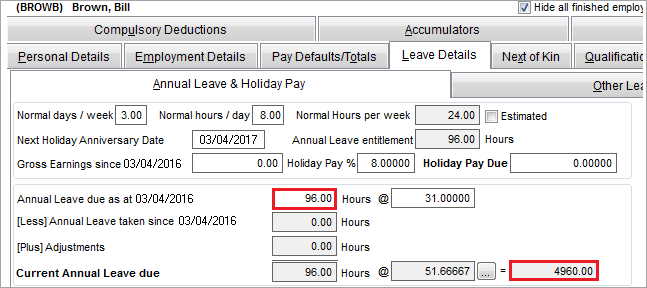In Bill's example above, changing the Annual Leave due as at to 96 hours, the Current Annual Leave Due value has corrected to \$4960.

UI Expand
title Additional adjustment (if reducing employee hours part way through the year)

If an employee's hours are reduced part way through the year, a pro rata manual adjustment will be required at the employee's Holiday Anniversary Date to take into account the period of the year their annual leave entitlement was greater.

To work this out, make a note of the amount of time the employee was at the increased/full time hours, e.g. 40 hours a week, so 160 hours a year for 5 months, so 160 / 12 = 13.3 x 5 (months) = 66.5 hours.

If the employee reduced their hours to 24 hours a week, this gives them an annual leave entitlement of 96 hours a year. But this amount would only be for 7 months (as the first 5 months, they worked 40 hours a week). So 96 hours / 12 (months) x 7 (months) = 56 hours.

Based on this calculation, an additional 26 hours needs to be included. This is because MYOB Payroll has automatically calculated 96 hours of annual leave (based on the new normal hours per week, which is 24 hours), however the employee is entitled to 122 hours of annual leave (66.5 + 56 = 122 hours).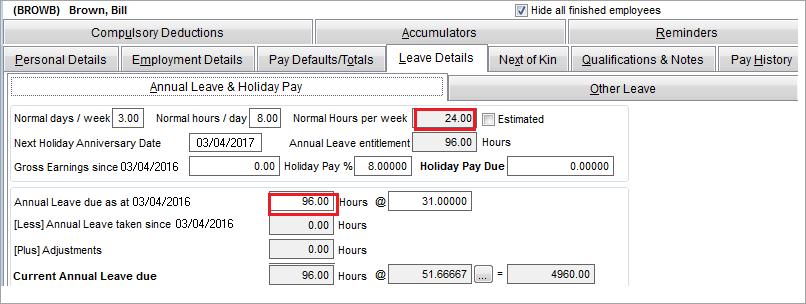Image Removed

In Bill's example above, the Annual Leave due as at is sitting at 96 hours as per his new normal hours. However, Bill is entitled to 122 hours (66.5 + 56 = 122), so this amount needs to be changed to show the correct annual leave.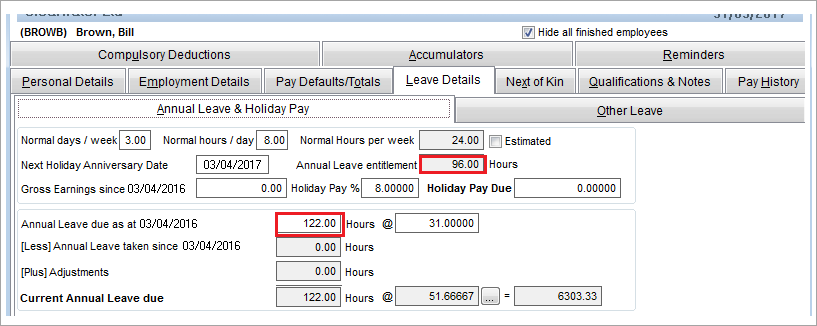Image Removed

HTML Wrap
width 15% col span_1_of_5

HTML Wrap
float left col span_1_of_5
Panelbox
name green Related topics